## Manually Convert a Floating-Point MATLAB Algorithm to Fixed Point

This example shows how to convert a floating-point algorithm to fixed point and then generate C code for the algorithm. The example uses the following best practices:

• Separate your algorithm from the test file.

• Prepare your algorithm for instrumentation and code generation.

• Manage data types and control bit growth.

• Separate data type definitions from algorithmic code by creating a table of data definitions.

For a complete list of best practices, see Manual Fixed-Point Conversion Best Practices.

### Separate Your Algorithm From the Test File

Write a MATLAB® function, `mysum`, that sums the elements of a vector.

```function y = mysum(x) y = 0; for n = 1:length(x) y = y + x(n); end end ```

Since you only need to convert the algorithmic portion to fixed-point, it is more efficient to structure your code so that the algorithm, in which you do the core processing, is separate from the test file.

### Write a Test Script

In the test file, create your inputs, call the algorithm, and plot the results.

1. Write a MATLAB script, `mysum_test`, that verifies the behavior of your algorithm using double data types.

```n = 10; rng default x = 2*rand(n,1)-1; % Algorithm y = mysum(x); % Verify results y_expected = sum(double(x)); err = double(y) - y_expected```

`rng default` puts the settings of the random number generator used by the rand function to its default value so that it produces the same random numbers as if you restarted MATLAB.

2. Run the test script.

`mysum_test`
```err = 0```

The results obtained using `mysum` match those obtained using the MATLAB `sum` function.

For more information, see Create a Test File.

### Prepare Algorithm for Instrumentation and Code Generation

In your algorithm, after the function signature, add the `%#codegen` compilation directive to indicate that you intend to instrument the algorithm and generate C code for it. Adding this directive instructs the MATLAB code analyzer to help you diagnose and fix violations that would result in errors during instrumentation and code generation.

```function y = mysum(x) %#codegen y = 0; for n = 1:length(x) y = y + x(n); end end ```

For this algorithm, the code analyzer indicator in the top right corner of the editor window remains green telling you that it has not detected any issues.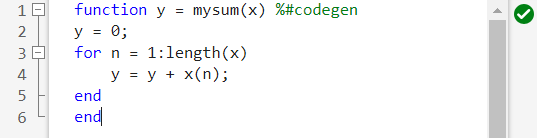For more information, see Prepare Your Algorithm for Code Acceleration or Code Generation.

### Generate C Code for Your Original Algorithm

Generate C code for the original algorithm to verify that the algorithm is suitable for code generation and to see the floating-point C code. Use the `codegen` (MATLAB Coder) function (requires MATLAB Coder™) to generate a C library.

1. Add the following line to the end of your test script to generate C code for `mysum`.

`codegen mysum -args {x} -config:lib -report`

2. Run the test script again.

MATLAB Coder generates C code for `mysum` function and provides a link to the code generation report.

3. Click the link to open the code generation report and view the generated C code for `mysum`.

```/* Function Definitions */ double mysum(const double x) { double y; int n; y = 0.0; for (n = 0; n < 10; n++) { y += x[n]; } return y; }```

Because C does not allow floating-point indices, the loop counter, `n`, is automatically declared as an integer type. You do not need to convert `n` to fixed point.

Input `x` and output `y` are declared as double.

### Manage Data Types and Control Bit Growth

Test Your Algorithm With Singles to Check for Type Mismatches

1. Modify your test file so that the data type of `x` is single.

```n = 10; rng default x = single(2*rand(n,1)-1); % Algorithm y = mysum(x); % Verify results y_expected = sum(double(x)); err = double(y) - y_expected codegen mysum -args {x} -config:lib -report```

2. Run the test script again.

`mysum_test`
```err = -4.4703e-08 ??? This assignment writes a 'single' value into a 'double' type. Code generation does not support changing types through assignment. Check preceding assignments or input type specifications for type mismatches.```

Code generation fails, reporting a data type mismatch on line ```y = y + x(n);```.

3. To view the error, open the report.

In the report, on the line `y = y + x(n)`, the report highlights the `y` on the left side of the assignment in red to indicate that there is an error. The issue is that `y` is declared as a double but is being assigned to a single. `y + x(n)` is the sum of a double and a single which is a single. If you place your cursor over variables and expressions in the report, you can see information about their types. Here, you can see that the expression, `y + x(n)` is a single.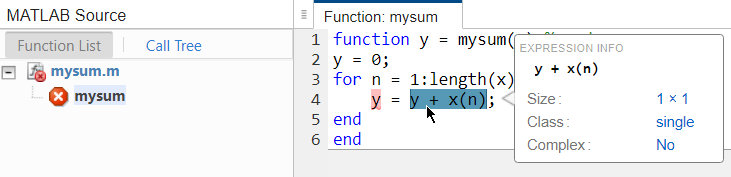4. To fix the type mismatch, update your algorithm to use subscripted assignment for the sum of elements. Change `y = y + x(n)` to ```y(:) = y + x(n)```.

```function y = mysum(x) %#codegen y = 0; for n = 1:length(x) y(:) = y + x(n); end end ```

Using subscripted assignment, you also prevent the bit growth which is the default behavior when you add fixed-point numbers. For more information, see Bit Growth. Preventing bit growth is important because you want to maintain your fixed-point types throughout your code. For more information, see Controlling Bit Growth.

5. Regenerate C code and open the code generation report. In the C code, the result is now cast to double to resolve the type mismatch.

### Build Instrumented Mex

Use the `buildInstrumentedMex` function to instrument your algorithm for logging minimum and maximum values of all named and intermediate variables. Use the `showInstrumentationResults` function to propose fixed-point data types based on these logged values. Later, you use these proposed fixed-point types to test your algorithm.

1. Update the test script:

1. After you declare `n`, add ```buildInstrumentedMex mySum —args {zeros(n,1)} -histogram```.

2. Change `x` back to double. Replace ```x = single(2*rand(n,1)-1);``` with `x = 2*rand(n,1)-1;`

3. Instead of calling the original algorithm, call the generated MEX function. Change `y = mysum(x)` to `y=mysum_mex(x)`.

4. After calling the MEX function, add ```showInstrumentationResults mysum_mex -defaultDT numerictype(1,16) -proposeFL```. The `-defaultDT numerictype(1,16) -proposeFL` flags indicate that you want to propose fraction lengths for a 16-bit word length.

Here is an updated test script.

```%% Build instrumented mex n = 10; buildInstrumentedMex mysum -args {zeros(n,1)} -histogram %% Test inputs rng default x = 2*rand(n,1)-1; % Algorithm y = mysum_mex(x); % Verify results showInstrumentationResults mysum_mex ... -defaultDT numerictype(1,16) -proposeFL y_expected = sum(double(x)); err = double(y) - y_expected %% Generate C code codegen mysum -args {x} -config:lib -report ```

2. Run the test script again.

The `showInstrumentationResults` function proposes data types and opens a report to display the results.

3. In the report, click the Variables tab. `showInstrumentationResults` proposes a fraction length of 13 for `y` and 15 for `x`.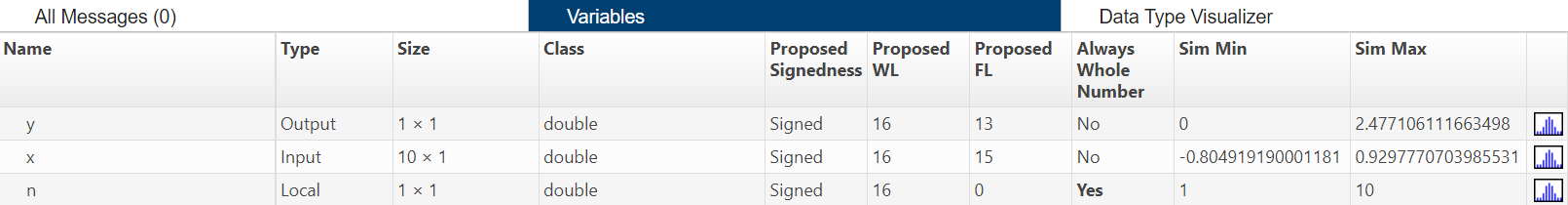In the report, you can:

• View the simulation minimum and maximum values for the input `x` and output `y`.

• View the proposed data types for `x` and `y`.

• View information for all variables, intermediate results, and expressions in your code.

To view this information, place your cursor over the variable or expression in the report.

• View the histogram data for `x` and `y` to help you identify any values that are outside range or below precision based on the current data type.

To view the histogram for a particular variable, click its histogram icon,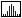.

### Separate Data Type Definitions From Algorithmic Code

Rather than manually modifying the algorithm to examine the behavior for each data type, separate the data type definitions from the algorithm.

Modify `mysum` so that it takes an input parameter, `T`, which is a structure that defines the data types of the input and output data. When `y` is first defined, use the `cast` function like syntax — `cast(x,'like',y)` — to cast `x` to the desired data type.

```function y = mysum(x,T) %#codegen y = cast(0,'like',T.y); for n = 1:length(x) y(:) = y + x(n); end end```

### Create a Table of Data Type Definitions

Write a function, `mytypes`, that defines the different data types that you want to use to test your algorithm. In your data types table, include double, single, and scaled double data types as well as the fixed-point data types proposed earlier. Before converting your algorithm to fixed point, it is good practice to:

• Test the connection between the data type definition table and your algorithm using doubles.

• Test the algorithm with singles to find data type mismatches and other problems.

• Run the algorithm using scaled doubles to check for overflows.

```function T = mytypes(dt) switch dt case 'double' T.x = double([]); T.y = double([]); case 'single' T.x = single([]); T.y = single([]); case 'fixed' T.x = fi([],true,16,15); T.y = fi([],true,16,13); case 'scaled' T.x = fi([],true,16,15,... 'DataType','ScaledDouble'); T.y = fi([],true,16,13,... 'DataType','ScaledDouble'); end end```

For more information, see Separate Data Type Definitions from Algorithm.

### Update Test Script to Use Types Table

Update the test script, `mysum_test`, to use the types table.

1. For the first run, check the connection between table and algorithm using doubles. Before you declare `n`, add ```T = mytypes('double');```

2. Update the call to `buildInstrumentedMex` to use the type of `T.x` specified in the data types table: ```buildInstrumentedMex mysum -args {zeros(n,1,'like',T.x),T} -histogram```

3. Cast `x` to use the type of `T.x` specified in the table: `x = cast(2*rand(n,1)-1,'like',T.x);`

4. Call the MEX function passing in `T`: ```y = mysum_mex(x,T);```

5. Call `codegen` passing in `T`: ```codegen mysum -args {x,T} -config:lib -report```

Here is the updated test script.

```%% Build instrumented mex T = mytypes('double'); n = 10; buildInstrumentedMex mysum ... -args {zeros(n,1,'like',T.x),T} -histogram %% Test inputs rng default x = cast(2*rand(n,1)-1,'like',T.x); % Algorithm y = mysum_mex(x,T); % Verify results showInstrumentationResults mysum_mex ... -defaultDT numerictype(1,16) -proposeFL y_expected = sum(double(x)); err = double(y) - y_expected %% Generate C code codegen mysum -args {x,T} -config:lib -report ```

6. Run the test script and click the link to open the code generation report.

The generated C code is the same as the code generated for the original algorithm. Because the variable `T` is used to specify the types and these types are constant at code generation time; `T` is not used at run time and does not appear in the generated code.

### Generate Fixed-Point Code

Update the test script to use the fixed-point types proposed earlier and view the generated C code.

1. Update the test script to use fixed-point types. Replace ```T = mytypes('double');``` with `T = mytypes('fixed');` and then save the script.

2. Run the test script and view the generated C code.

This version of C code is not very efficient; it contains a lot of overflow handling. The next step is to optimize the data types to avoid overflows.

### Optimize Data Types

#### Use Scaled Doubles to Detect Overflow

Scaled doubles are a hybrid between floating-point and fixed-point numbers. Fixed-Point Designer™ stores them as doubles with the scaling, sign, and word length information retained. Because all the arithmetic is performed in double-precision, you can see any overflows that occur.

1. Update the test script to use scaled doubles. Replace ```T = mytypes('fixed');``` with `T = mytypes('scaled');`

2. Run the test script again.

The test runs using scaled doubles and displays the report. No overflows are detected.

So far, you’ve run the test script using random inputs which means that it is unlikely that the test has exercised the full operating range of the algorithm.

3. Find the full range of the input.

`range(T.x)`
```-1.000000000000000 0.999969482421875 DataTypeMode: Fixed-point: binary point scaling Signedness: Signed WordLength: 16 FractionLength: 15```

4. Update the script to test the negative edge case. Run `mysum_mex` with the original random input and with an input that tests the full range and aggregate the results.

```%% Build instrumented mex T = mytypes('scaled'); n = 10; buildInstrumentedMex mysum ... -args {zeros(n,1,'like',T.x),T} -histogram %% Test inputs rng default x = cast(2*rand(n,1)-1,'like',T.x); y = mysum_mex(x,T); % Run once with this set of inputs y_expected = sum(double(x)); err = double(y) - y_expected % Run again with this set of inputs. The logs will aggregate. x = -ones(n,1,'like',T.x); y = mysum_mex(x,T); y_expected = sum(double(x)); err = double(y) - y_expected % Verify results showInstrumentationResults mysum_mex ... -defaultDT numerictype(1,16) -proposeFL y_expected = sum(double(x)); err = double(y) - y_expected %% Generate C code codegen mysum -args {x,T} -config:lib -report ```

5. Run the test script again.

The test runs and `y` overflows the range of the fixed-point data type. `showInstrumentationResults` proposes a new fraction length of 11 for `y`.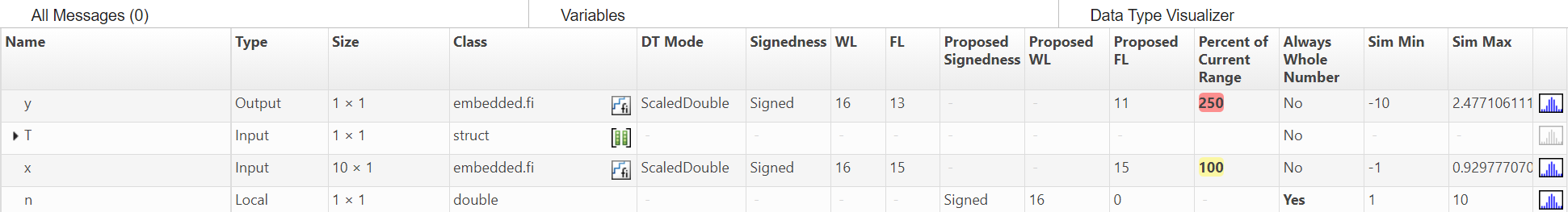6. Update the test script to use scaled doubles with the new proposed type for `y`. In `myTypes.m`, for the `'scaled'` case, ``` T.y = fi([],true,16,11,'DataType','ScaledDouble')```

7. Rerun the test script.

There are now no overflows.

#### Generate Code for the Proposed Fixed-Point Type

Update the data types table to use the proposed fixed-point type and generate code.

1. In `myTypes.m`, for the `'fixed'` case, `T.y = fi([],true,16,11)`

2. Update the test script, `mysum_test`, to use ```T = mytypes('fixed');```

3. Run the test script and then click the View Report link to view the generated C code.

```short mysum(const short x) { short y; int n; int i; int i1; int i2; int i3; y = 0; for (n = 0; n < 10; n++) { i = y << 4; i1 = x[n]; if ((i & 1048576) != 0) { i2 = i | -1048576; } else { i2 = i & 1048575; } if ((i1 & 1048576) != 0) { i3 = i1 | -1048576; } else { i3 = i1 & 1048575; } i = i2 + i3; if ((i & 1048576) != 0) { i |= -1048576; } else { i &= 1048575; } i = (i + 8) >> 4; if (i > 32767) { i = 32767; } else { if (i < -32768) { i = -32768; } } y = (short)i; } return y; }```

By default, `fi` arithmetic uses saturation on overflow and nearest rounding which results in inefficient code.

#### Modify fimath Settings

To make the generated code more efficient, use fixed-point math (`fimath`) settings that are more appropriate for C code generation: wrap on overflow and floor rounding.

1. In `myTypes.m`, add a `'fixed2'` case:

``` case 'fixed2' F = fimath('RoundingMethod', 'Floor', ... 'OverflowAction', 'Wrap', ... 'ProductMode', 'FullPrecision', ... 'SumMode', 'KeepLSB', ... 'SumWordLength', 32, ... 'CastBeforeSum', true); T.x = fi([],true,16,15,F); T.y = fi([],true,16,11,F); ```

Tip

Instead of manually entering `fimath` properties, you can use the MATLAB Editor Insert fimath option. For more information, see Building fimath Object Constructors in a GUI.

2. Update the test script to use `'fixed2'`, run the script, and then view the generated C code.

```short mysum(const short x) { short y; int n; y = 0; for (n = 0; n < 10; n++) { y = (short)(((y << 4) + x[n]) >> 4); } return y; }```

The generated code is more efficient, but `y` is shifted to align with `x` and loses 4 bits of precision.

3. To fix this precision loss, update the word length of `y` to 32 bits and keep 15 bits of precision to align with `x`.

In `myTypes.m`, add a `'fixed32'` case:

``` case 'fixed32' F = fimath('RoundingMethod', 'Floor', ... 'OverflowAction', 'Wrap', ... 'ProductMode', 'FullPrecision', ... 'SumMode', 'KeepLSB', ... 'SumWordLength', 32, ... 'CastBeforeSum', true); T.x = fi([],true,16,15,F); T.y = fi([],true,32,15,F); ```

4. Update the test script to use `'fixed32'` and run the script to generate code again.

Now, the generated code is very efficient.

```int mysum(const short x) { int y; int n; y = 0; for (n = 0; n < 10; n++) { y += x[n]; } return y; }```